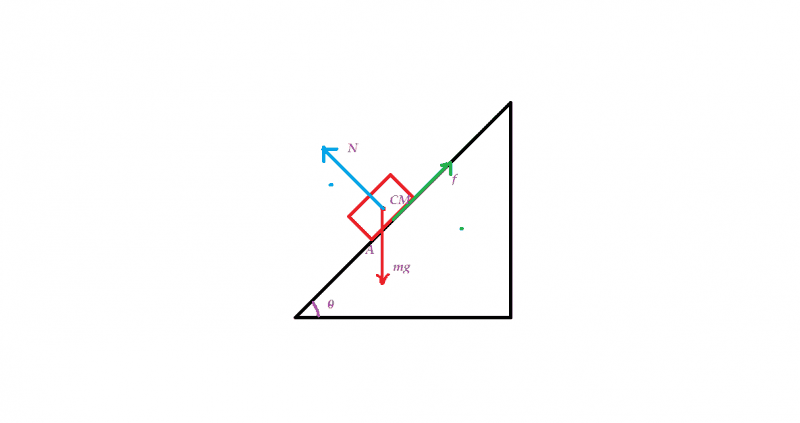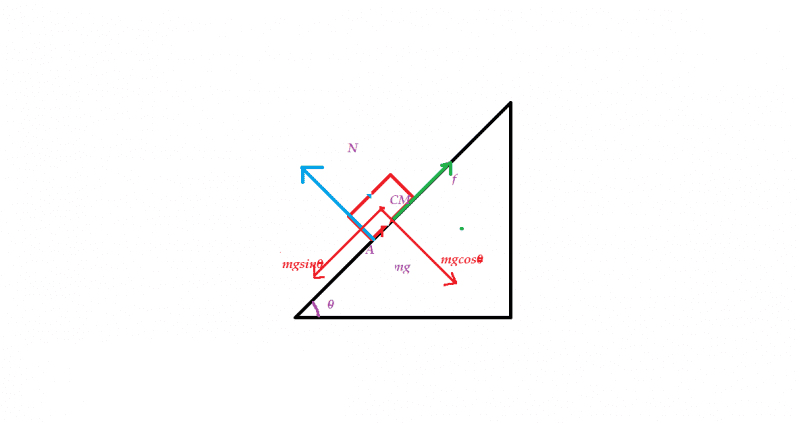# Block Toppling on an incline

## Homework Statement

A block of base ##10cm \times 10cm## and height ##15cm## is kept on an incline plane. The coefficient of friction between them is ##\sqrt { 3 } ##. The inclination of the incline plain is ##\theta##. Find the value of theta for which the block begins to topple.

## Homework Equations

1)##\tau =I\alpha ##
2)For equilibrium ##\sum { \tau } =0##

## The Attempt at a Solution

I [/B]tried to make free body diagram of the block.As the block begins to topple the I think at that moment point A can be treated as Instantaneous Axis of rotation. Can it be?

When the block begins to topple, does the Normal force on block would shift to point A?

If so then, if I take the moment of forces acting on the block then torque of force ##N## and ##f## about A will be ##0##. But that's not correct.
How should I proceed. Sorry if I am missing something very common.

Last edited:

BvU
Homework Helper
In your post I miss the relevant equations....

First thing that strikes me in the picture is that the block isn't resting on its base...

Your question about the action line of the normal force is interesting; intuitively I'm with you, but not quite certain. So perhaps we can try to make it irrelevant.

In your post I miss the relevant equations....

First thing that strikes me in the picture is that the block isn't resting on its base...

Your question about the action line of the normal force is interesting; intuitively I'm with you, but not quite certain. So perhaps we can try to make it irrelevant.

I have added two equations in my questions. Please have a look over them.

Could you please tell me how to proceed.

does the Normal force on block would shift to point A?

Very good question. To answer it, consider a more general problem: at which point should the normal force be applied for the block to be in equilibrium on an incline?

ehild
Homework Helper
Analyse the situation when the block is raised up a little bit from the slope, so that it touches it with the bottom edge only. Releasing, it either returns to its stable position, or topples over.
In that raised --up position, the normal force acts et the edge.

BvU
Homework Helper
Time to write down some of the torques around point A ....

haruspex
Homework Helper
Gold Member
2020 Award
When the block begins to topple, does the Normal force on block would shift to point A?

If so then, if I take the moment of forces acting on the block then torque of force ##N## and ##f## about A will be ##0##. But that's not correct.
Why do you say it is not correct?

Is the value of ##\theta## 33.69?

haruspex
Homework Helper
Gold Member
2020 Award
Is the value of ##\theta## 33.69?
Yes, except that you had to make an assumption to arrive at that. Think about the given information you have not used.

Yes, except that you had to make an assumption to arrive at that. Think about the given information you have not used.

I have not used value of friction because the frictional force passes through the point about which I have calculated torque. How should I include that?
Should I calculate torque about CoM of the cube?

ehild
Homework Helper
The coefficient of friction is quite big. What do you think, can the block slide on the slope ?

•Satvik Pandey
The coefficient of friction is quite big. What do you think, can the block slide on the slope ?

Well that depends on the value of ##\theta##. It will not slide unless the value of ##\theta## is equal to the 60.

If the question asks us to to find the minimum value of ##\mu## (coefficient of static friction) required for block to topple for given ##\theta## then how should I approach to that question.
If I consider moment of forces about point A(please see figure in #post1) then I would not be able to get an expression involving the ##\mu##. Should I consider torques about the CoM of the box?

BvU
Homework Helper
OK, so you found 33.69 degrees, or rather ##\arctan{10\over 15}## as the angle at which the thing topples -- provided the friction force is sufficient.
Since this is smaller than 60 degrees (or rather, ##\arctan\sqrt 3 ##), the block will not slide but topple, so that's OK.

At an angle ##\theta## it will slide when the friction force ## \mu_s mg \cos\theta ## is less than ## mg \sin \theta##, in other words, ## \mu_s \lt \ ?\ ##

If I consider moment of forces about point A(please see figure in #post1) then I would not be able to get an expression involving the ##\mu## . Should I consider torques about the CoM of the box?
Not necessary: all you want there is that it doesn't slide and that is about forces, not torques.

haruspex
Homework Helper
Gold Member
2020 Award
If I consider moment of forces about point A(please see figure in #post1) then I would not be able to get an expression involving the ##\mu##.
Quite so, but you're trying to answer whether it would slide, so you need to look at linear forces, not moments.

the thing topples -- provided the friction force is sufficient

Why is the latter required? As far as I can see, if the block topples, it shall do so even if friction is absent.

haruspex
Homework Helper
Gold Member
2020 Award
Why is the latter required? As far as I can see, if the block topples, it shall do so even if friction is absent.
Without friction, it will slide down the plane. What happens when it gets to the bottom we don't know. Maybe it slides right off, so won't topple.

BvU
Homework Helper
@voko: strong point. I imagine a gradual increase of theta until ... etc. There is no solid base for that perception, I agree. However, with ##\theta > \arctan{10\over 15}## and no friction, where can the torque to rotate around the c.o.m. come from ?

Now what about post 14 ? Makes things murky for me: no toppling unless ##\theta > \arctan{10\over 15}## and ##\mu## is irrelevant then ? Doesn't feel good.

Haru is on the same line I think.

Without friction, it will slide down the plane.

Even without friction, the normal force and the weight can produce a non-zero couple when the angle is sufficiently great.

BvU
Homework Helper
Don't see a rotation around the c.o.m. coming up ...

Don't see a rotation around the c.o.m. coming up ...

BvU
Homework Helper
That's indeed the key one. What's the line of action for the normal force. My idea: any normal force that would cause a rotation is immediately offset by a shift of the line of action. If N acts at Satvik's point A, that represents a clockwise torque (in combination with ##mg\cos\theta\, ##). All that torque can do is shift the line of action of N upwards.

Would love to trade this gut feeling for a thorough bit of physics, though ... :)

In equilibrium, the sum of all moments is zero. At some point X within the base of the block, the normal force is exerted. Taking moments about X, the moments of the normal force and friction are zero. So the moment of gravity must also be zero. Clearly this is possible only when X is at the intersection of the vertical line from the C. o. M. with the base (this is a well known "toppling" stability criterion). Equally clear is that at certain angles this is no longer possible, because the vertical line from the C. o. M. will be outside the base, regardless of friction.

•Satvik PandeyI found the answer in this way

Finding moments of forces about point A

Block start toppling if

##7.5mg sin\theta=5mg cos\theta##

or ##tan\theta=\frac{5}{7.5}##

In this there was no use of friction force.

So can we say that even if the friction force is zero the block will topple.

Voko is this what you were saying in #post 19?

What will happen if ##\theta >60##. Will it topple and slide together?